# NCERT Solutions for Class 9 Maths Exercise 4.4## myCBSEguide App

CBSE, NCERT, JEE Main, NEET-UG, NDA, Exam Papers, Question Bank, NCERT Solutions, Exemplars, Revision Notes, Free Videos, MCQ Tests & more.

NCERT solutions for Class 9 Maths Linear Equations in Two Variables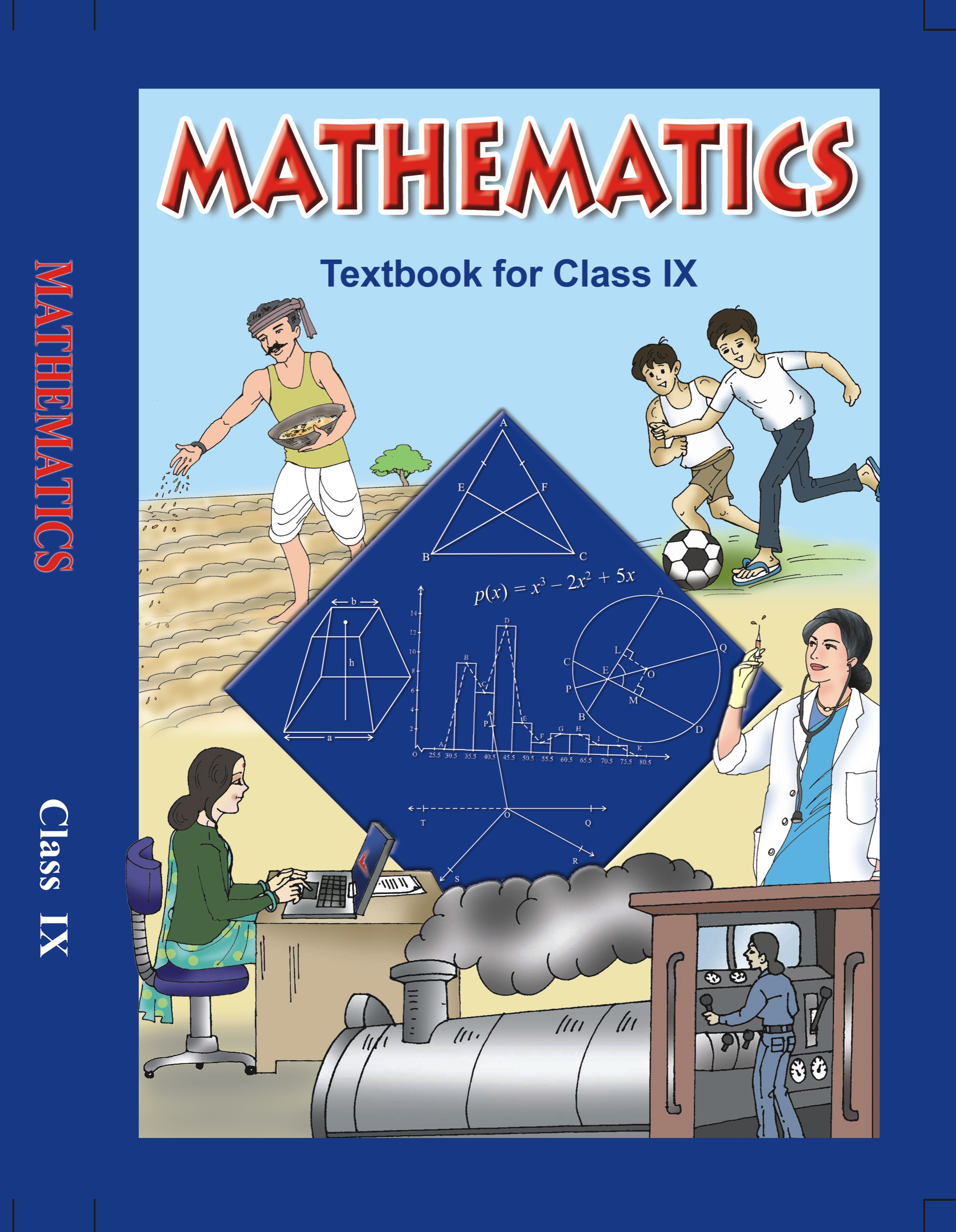## NCERT Solutions for Class 9 Mathematics Linear Equations in Two Variables

###### 1. Give the geometric representation of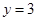as an equation

(i) In one variable

(ii) In two variables

Ans. (i) We need to represent the linear equationgeometrically in one variable.

We can conclude that in one variable, the geometric representation of the linear equationwill be same as representing the number 3 on a number line.

Given below is the representation of number 3 on the number line.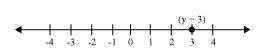###### (ii) We need to represent the linear equationgeometrically in two variables.

We know that the linear equationcan also be written as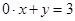.

We can conclude that in two variables, the geometric representation of the linear equationwill be same as representing the graph of linear equation.

Given below is the representation of the linear equationon a graph.

We can optionally consider the given below table for plotting the linear equationon the graph.

 X 1 0 y 3 3
######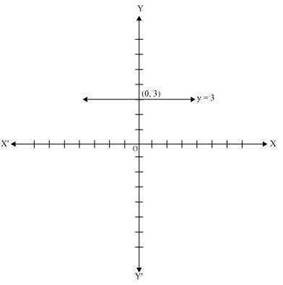NCERT Solutions for Class 9 Maths Exercise 4.4

###### 2. Give the geometric representations of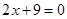as an equation

(i) In one variable

(ii) In two variables

Ans. (i) We need to represent the linear equationgeometrically in one variable.

We know that the linear equationcan also be written as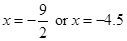.

We can conclude that in one variable, the geometric representation of the linear equationwill be same as representing the number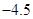on a number line.

Given below is the representation of number 3 on the number line.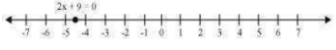###### (ii) We need to represent the linear equationgeometrically in two variables.

We know that the linear equationcan also be written as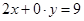.

We can conclude that in two variables, the geometric representation of the linear equationwill be same as representing the graph of linear equation.

Given below is the representation of the linear equationon a graph.

We can optionally consider the given below table for plotting the linear equationon the graph.

 X 1 0 y 4.5 4.5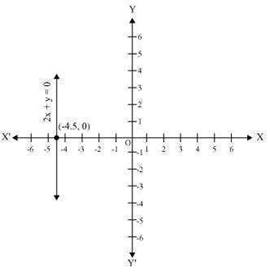## NCERT Solutions for Class 9 Maths Exercise 4.4

NCERT Solutions for Class 9 Maths PDF (Download) Free from myCBSEguide app and myCBSEguide website. Ncert solution class 9 Maths includes text book solutions from Mathematics Book. NCERT Solutions for CBSE Class 9 Maths have total 15 chapters. 9 Maths NCERT Solutions in PDF for free Download on our website. Ncert Maths class 9 solutions PDF and Maths ncert class 9 PDF solutions with latest modifications and as per the latest CBSE syllabus are only available in myCBSEguide.

## CBSE app for Class 9

To download NCERT Solutions for Class 9 Maths, Computer Science, Home Science,Hindi ,English, Social Science do check myCBSEguide app or website. myCBSEguide provides sample papers with solution, test papers for chapter-wise practice, NCERT solutions, NCERT Exemplar solutions, quick revision notes for ready reference, CBSE guess papers and CBSE important question papers. Sample Paper all are made available through the best app for CBSE students and myCBSEguide website.### 5 thoughts on “NCERT Solutions for Class 9 Maths Exercise 4.4”

1. Excellent service it helps us in studying ??

2. Thanks